# Monthly Archives: February 2018

## Digging Deeper into … Directed Numbers (5th & 6th classes)

Category : Uncategorized

In Operation Maths for 5th Class the children are formally introduced to the concept of directed numbers (from the strand of algebra) and are enabled to:

• Explore and identify directed numbers, i.e. numbers above and below zero, including zero
• Describe and record directed numbers as positive or negative
• Use directed numbers to represent real-life situations
• Compare and order directed numbers
• Solve problems involving directed numbers.

In Operation Maths for 6th class, this knowledge and understanding is revised and extended to include the addition of positive and negative numbers.

Directed numbers (formally known as integers, the set of positive and negative whole numbers including zero) can be a very difficult concept for some children for a number of reasons:

• Being able to explore numbers less than zero requires a solid understanding of zero, an abstract concept in itself since it indicates the absence of something
• Many children find it difficult to comprehend how something can be less than zero. Therefore, when ordering it can be difficult for many children to appreciate that 0 is greater than -1, -2, etc.
• Operations involving integers are particularly troublesome as having similar signs so close together can be very confusing.

Use real-life contexts

In fifth class, since this is the first time that the children have been formally introduced to negative numbers, it is essential that this happens through reference to real-life contexts, e.g. temperature, buttons in a lift, goal difference in soccer league tables, depths in a swimming pool, or any other context with which the children can readily identify. Other real-life contexts such as bank account balances, elevations, etc., can also be used.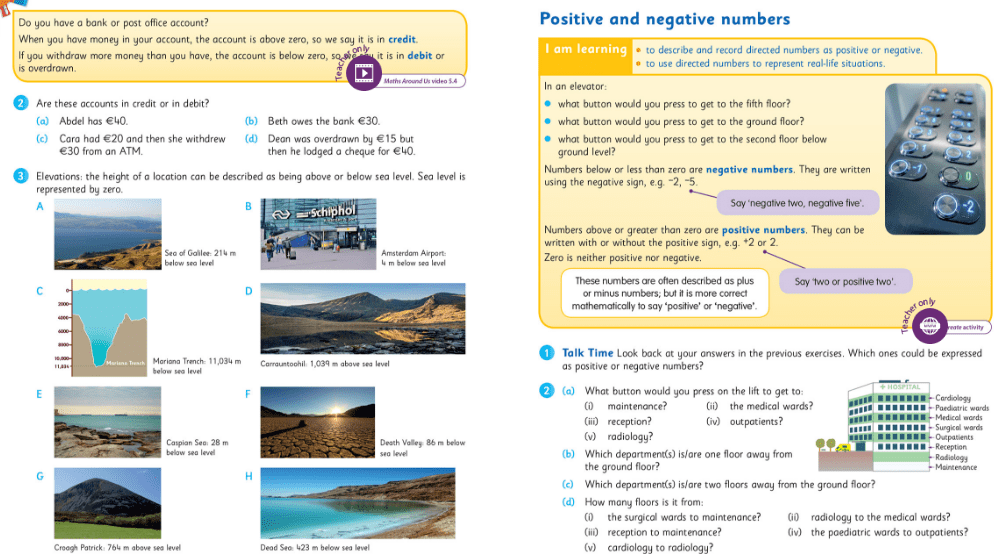### Use ‘positive’ and ‘negative’ rather than ‘plus’ and ‘minus’

Whilst real-life contexts are useful, they can also be confusing, particularly when plus and minus are used to describe directed numbers. Insist that the children use the language of positive and negative, since that is more correct mathematically. Using positive and negative can also reduce complications in future classes when calculating with directed numbers, e.g. avoiding having to say three minus minus five for 3-(-5). This is particularly important when the children progress to adding positive and negative numbers: for example (–3) + (+9) should be read as ‘negative three add/plus positive nine’ rather than ‘minus 3 plus plus 9’. It is also important that the children recognise that positive numbers can be written either with, or without, the positive sign, therefore we can assume that any number without a sign is positive.

To further reduce complications with written forms of directed numbers, raised signs should be used for the positive and negative (as shown in image below) and brackets should be used to make it easier for the child to distinguish between the operations signs (i.e. + and -) and the directed numbers signs.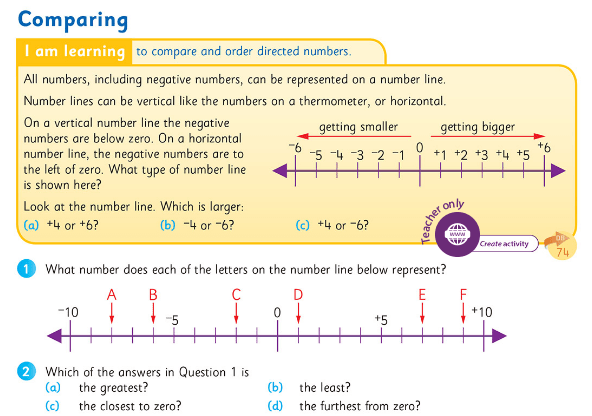### Representing directed numbers

Since negative numbers are so abstract, it is vital that opportunities to represent them concretely and pictorially are maximised. Many children consider only the cardinality of numbers, i.e. that they represent the number of objects in a set, whereas understanding of directed numbers relies on understanding the ordinality of numbers; this is why visual representations are so vital.

One way to do this, is to use a counting stick, if available. Start with the counting stick in a vertical position, asking the children that if the centre line is now representing zero, can they identify the points above and below that?

• Initially describe the positions as “one above zero”, “two below zero” etc
• Then, when appropriate, introduce positions as “positive three”, “negative four” etc
• Development: if each interval now represents two/ten/five as opposed to one, how does this change the identity of the positions?
• Finally, move the counting stick into a horizontal position, so that the negative numbers are now to the left of the centre/zero and the positive to the right (as the children see it).

Operation Maths users can also use the counting stick eManipulative to model the counting stick in a horizontal position, as shown below (Hint: zoom the screen in to 150% to view the numbers better).Number lines are also very useful. When using number lines (or a counting stick), emphasize that numbers get smaller in value as you move above/to the left of zero, and larger as you move below/to the right of zero . If possible, have a number line that includes negative numbers on permanent display in the classroom.

The Operation Maths MWBs can also be used to create a dynamic number line for ordering activities. For example, ask individual children to write a specific integer on their MWB. Then ask a group of children, each with different integers,  to stand out and put their MWBs in order. These numbers could even be hung from a ‘washing line’ using clothes pegs.

In sixth class, to better illustrate the processes involved with the addition of integers, teachers are encouraged to use the positive and negative chips, that accompany the sixth class books as part of the free ancillary resources (they’re on the same sheet as the images of the base ten block for modelling decimal numbers).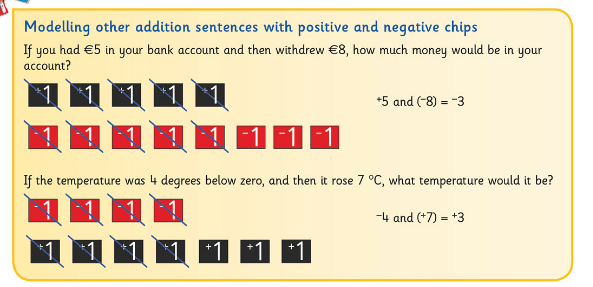The teacher can also use the positive and negative chips built into the Sorting eManipulative to model calculations on the class IWB, as shown below (the positive and negative chips are located at the end of the numbers tab).The children can then progress to using number lines to both reinforce their understanding of addition with integers and extend it to bigger numbers. A physical number line that the children can walk along would be ideal initially, as this suits the kinaesthetic learners and the bigger nature makes it easier for teachers to assess the children’s ability to use the number line before they move to smaller scale number lines in books and copies.### Online Interactive Resources

• Operation Maths users don’t forget to use the Counting Stick eManipulative as mentioned earlier and to check out the Maths Around Us video for this topic on Edco Learning. Check out the first page of the Directed Numbers chapter in the Pupils Books for a quick synopsis of the suggested digital resources and then refer to the Directed Numbers chapter in the TRB for more detailed information.
• That Quiz: Inequalities for comparing whole numbers, Arithmetic for addition calculations involving directed numbers and Number line for identifying numbers by their position. In each of these activities there is the option to include negative numbers along with positive; just make sure the the “negative” option is selected on the left hand side.
• Practice placing positive and negative integers in the correct place on an empty number line
• Temperature change: Activity to calculate the temperature change from Day 1 to Day 2.
• Assortment of integer games from Topmarks,  Sheppard Software and Free Training Tutorial
• Check out this Pinterest Board of Algebra ideas

## Digging Deeper into … Length (all classes)

Category : Uncategorized

### Initial exploration of Length

Initial experiences of length in the infant classes should occur through exploration, discussion, and use of appropriate vocabulary eg long/short, tall/short, wide/narrow, longer, shorter, wider than etc. The children should also be enabled to sort, compare and order objects according to length or height.The Aistear Play suggestions in the Operation Maths TRBs for Junior and Senior Infants (see example above) provide very useful ideas that can be used as the basis for purposeful exploration and discussion, for example, in the case of the garden centre-themed suggestions above:

• What plant/tree is taller/shorter?
• What gardening tool is longer/shorter?
• What plant pot/row of plants is wider/narrower?

Concrete-based exploration should follow and initial questions and discussion, to include direct comparison of the length of two objects, labelling them as long/short and/or longer than/shorter than etc. This can then progress to incorporate a direct comparison of the length of three or more objects, to now also include the labels longest/shortest. It is important at this stage that the children realise that if A is shorter than B and B is shorter than C, then, without further direct comparisons, we know that A is shorter than C, that A is the shortest of all three and C is the longest. This is a very important concept for the children to grasp.

### Non-standard and standard units

Children in senior infants should begin to estimate and measure length using non-standard units of measure, such as lollipop sticks, straws, pencils etc. Non-standard units are specifically chosen, as opposed to the standard measures of metres and centimetres, as most objects that the children will choose to measure will be longer than 10cm, therefore outside the number limit of senior infants. Furthermore, the metre is almost too big a unit for them to work with at this stage, since the heights of many children in senior infants would be less than this. It’s difficult to develop a sense of a metre when it’s bigger than yourself! Whereas lollipops, straws etc are not too big nor too small for their hands and easier to work with. The children could also use a whole ruler as a non-standard unit in itself e.g. “the table is three rulers long”.

When the children have had some experiences measuring they should then be afforded the opportunity to choose which instrument is most appropriate to measure the length/height of various items. In this way, they start developing the notion that while many approaches can be taken, some are more efficient than others, and the most efficient approach will also depend on the target object being measured. This is the same as the Operation Maths approach to operations throughout the classes; there can be many approaches and some are more efficient than others, depending on the numbers/operations involved.  The aim is for the children to become accurate, efficient and flexible thinkers.

Children in first and second classes should also be afforded the opportunity to explore non-standard units of length prior to being introduced to the standard units. Historical non-standard units can be introduced also eg spans, digits, cubits, strides etc. Work using these measures will not only encourage the children to appreciate the need for standard units, but once they are introduced to the metre (first class) and the centimetre (second class) they should also try to identify which non-standard units are closest to the standard units, eg the child’s stride or arm span is often close to a metre and their digit is close to a centimetre (quick investigation for second class: the width of which of your fingers is closest to a centimetre?). Connecting these standard units of measure back to the children themselves helps them identify with the measure and helps them internalise a sense of its size.

### Estimating & measuring

The children should always be encouraged to estimate before measuring. And rather than estimating the length of A, B, C and D before measuring A, B, C and D, it would be better if the children estimated the length of A and then measured the length of A,  estimated the length of B and then measured the length of B and so on. Thus, they can reflect on the reasonableness of their original estimate each time and use this to refine their next estimate so that it might be more accurate.  As mentioned previously, the child’s ability to connect standard units of length to themselves, not only helps them internalise a sense of length, but to also use this sense to produce more accurate estimates.

While the children begin using cm rulers in second class to measure length, they can still struggle to measure objects accurately in both this class and higher classes. Videos, such as the one below can be a useful way to demonstrate this skill to the whole class.

When children are performing tasks that require them to measure longer lengths (eg of the blackboard, the length of the room etc) using rulers or metre sticks, remind them to make sure that there are no gaps between their measuring instruments, that they keep them in a straight line and that they do not overlap. It can also be useful, if available, to use two metre sticks/rulers together so that once the second one is placed at the end of the first, the first ruler can then be moved to the end of the second ruler and so on.

### Renaming units of length

From third class on, the children will be expected to rename units of length appropriate to their class level. While changing 136 cm to 1m 36cm or 1.36m will typically be done correctly, converting figures which require zero as a placeholder (eg 1m 5cm, 2.4m ) are quite often problematic and can reveal an underlying gap in understanding, that is not revealed by the more obvious measures. In these cases, the children should be encouraged to return to the concrete and pictorial experiences as a way of checking the reasonableness of their answers, eg:

• “2.4m equals 240cm because 1m is 100cm (point to an actual metre stick) and then 2m is 200 cm and .4 is nearly .5 which is half of a metre which is almost 50 cm”
• “1m 5cm…well 1m is 100cm and then there’s 5cm more so it’s 100 plus 5, which is 105cm.

T-charts, one of the three key visual strategies for problem-solving used throughout Operation Maths, can be very useful when renaming units of length, as can be seen below.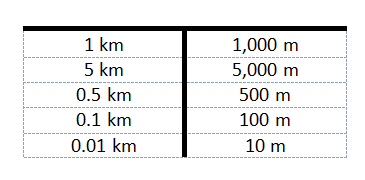### Perimeter and area

In Operation Maths, perimeter is deliberately taught separately from area; these topics are better taught independently because children can often confuse the concepts and processes of measuring area and perimeter.

Rather than introducing perimeter via a formula, it is critical initially that the children understand perimeter as the distance around the edge of a 2D shape and that they should actually measure around various 2D shapes as a way to identify the perimeter. Then, as they begin to look for more efficient ways to do this, they should be encouraged to discover and explain how they might calculate the perimeter of various types of shapes.

### Scale drawings

Scale drawing is introduced in sixth class. Encourage the children to experiment with drawing rooms, houses or gardens to scale. This may be integrated with map reading or making maps in geography.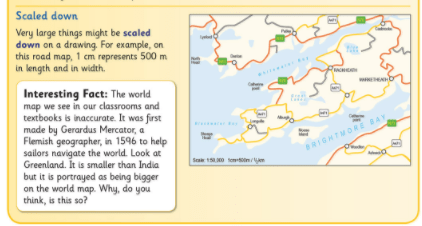T-charts, once again, can be very useful when exploring scale. For example,  see how a t-chart can be used to help answer the question, below: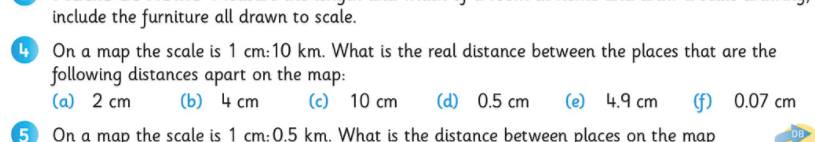When the children are making scale drawings themselves, or when they are solving problems such as those above,  it can be useful to use a t-chart to set out some benchmarks measures for reference: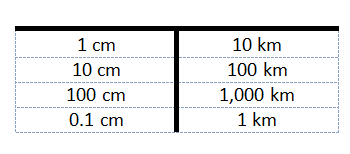A fun way to extend scale drawings, and to integrate maths with visual arts, is to do scale drawings of a simple cartoon or line drawing, with the finished scale being larger than the original eg 1:4. This slideplayer presentation could be used for inspiration.